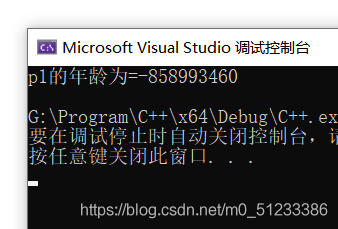# C++ this指針和空指針的具體使用

C++通過提供特殊的對象指針,this指針，解決上述問題。this指針指向被調用的成員函數所屬的對象

this指針是隱含每一個非靜態成員函數內的—種指針
this指針不需要定義，直接使用即可

this指針的用途:

• 當形參和成員變量同名時，可用this指針來區分
• 在類的非靜態成員函數中返回對象本身，可使用return this## 一、this指針

### 1 this指針解決名稱沖突

```#include <iostream>
using namespace std;
class Person
{
public:
Person(int age)
{
age = age;
}
int age;

};
//1 解決名稱沖突
void test1()
{
Person p1(18);
cout << "p1的年齡為=" << p1.age << endl;
}
int main()
{
test1();
return 0;
}
``````class Person
{
public:
Person(int age)
{
m_Age = age;
}
int m_Age;

};
```this指針指向 被調用的成員函數 所屬對象

```class Person
{
public:
//1 解決名稱沖突
Person(int age)
{
//this指針指向 被調用的成員函數 所屬對象
this->age = age;
}
int age;

};
```### 2 返回對象本身用*this

```class Person
{
public:
//1 解決名稱沖突
Person(int age)
{
//this指針指向 被調用的成員函數 所屬對象
this->age = age;
}

//2 返回對象本身用*this
{
this->age += p.age;
}

int age;

};
//1 解決名稱沖突
void test1()
{
Person p1(18);
cout << "p1的年齡為=" << p1.age << endl;

//2 返回對象本身用*this
Person p2(10);
cout << "p2的年齡為=" << p2.age << endl;
}
``````//2 返回對象本身用*this
{
//this指向p2的指針，而*this指向的就是p2這個對象的本體
this->age += p.age;
return *this;
}
```

```#include <iostream>
#include<string>
using namespace std;

//1 解決名稱沖突

//2 返回對象本身用*this

class Person
{
public:
//1 解決名稱沖突
Person(int age)
{
//this指針指向 被調用的成員函數 所屬對象
this->age = age;
}

//2 返回對象本身用*this
{
//this指向p2的指針，而*this指向的就是p2這個對象的本體
this->age += p.age;
return *this;
}

int age;

};
//1 解決名稱沖突
void test1()
{
Person p1(18);
cout << "p1的年齡為=" << p1.age << endl;

//2 返回對象本身用*this
Person p2(10);
cout << "p2的年齡為=" << p2.age << endl;
}

int main()
{
test1();
return 0;
}
```## 二、空指針調用成員函數

C++中空指針也是可以調用成員函數的，但是也要註意有沒有用到this指針

```#include<iostream>
using namespace std;

//空指針調用成員函數

class Person
{
public:
void showClassName()
{
cout << "This is Person class" << endl;
}

void showPersonAge()
{
//報錯原因是因為傳入的是空指針
cout << "age=" <<m_Age<< endl;
}
int m_Age;
};

void test1()
{
Person* p = NULL;
p->showClassName();
//	p->showPersonAge();//報錯原因是因為傳入的是空指針
}

int main()
{
test1();
return 0;
}
```//報錯原因是因為傳入的是空指針

if(this==NULL) return; //解決空指針出錯

```void showPersonAge()
{
//報錯原因是因為傳入的是空指針

if(this==NULL)  return; //解決空指針出錯

cout << "age=" <<m_Age<< endl;
}
```

```#include<iostream>
using namespace std;

//空指針調用成員函數

class Person
{
public:
void showClassName()
{
cout << "This is Person class" << endl;
}

void showPersonAge()
{
//報錯原因是因為傳入的是空指針

if(this==NULL)  return; //解決空指針出錯

cout << "age=" <<m_Age<< endl;
}
int m_Age;
};

void test1()
{
Person* p = NULL;
p->showClassName();
p->showPersonAge();//報錯原因是因為傳入的是空指針
}

int main()
{
test1();
return 0;
}
```## 三、const修飾成員函數

·成員函數後加const後我們稱為這個函數為常函數
.常函數內不可以修改成員屬性
·成員屬性聲明時加關鍵字mutable後，在常函數中依然可以修改

·聲明對象前加const稱該對象為常對象
·常對象隻能調用常函數mutable int m_B;//特殊變量，即使在常函數中，也可修飾這個值，加關鍵字mutable

```//常函數
class Person
{
public:
//this指針的本質是指針常量，指針的指向是不可以修改的
//const Person *const this;
//在成員函數後面加const，修飾的是this指向，讓指針指向的值也不可以修改
void showPerson() const
{
//	m_A = 100; //常函數內不可以修改成員屬性
//	this->m_A = 100;
//	this = NULL;
m_B = 100; //成員屬性聲明時加關鍵字mutable，在常函數中才可以修改
}

int m_A;
mutable int m_B;//特殊變量，即使在常函數中，也可修飾這個值，加關鍵字mutable
};
```

const Person p;//在對象前加const變常對象

```//常對象
void test2()
{
const Person p;//在對象前加const變常對象
//	p.m_A = 100;//報錯
p.m_B = 100;//m_B是特殊值，在常對象下也可以修改

//常對象隻能調用常函數
p.showPerson();
//	p.func();//常對象不可以調用普通成員函數，因為普通成員函數可以修改屬性
}
```

```#include<iostream>
using namespace std;

//常函數
//常對象

class Person
{
public:
//this指針的本質是指針常量，指針的指向是不可以修改的
//const Person *const this;
//在成員函數後面加const，修飾的是this指向，讓指針指向的值也不可以修改
void showPerson() const
{
//	m_A = 100; //常函數內不可以修改成員屬性
//	this->m_A = 100;
//	this = NULL;
m_B = 100; //成員屬性聲明時加關鍵字mutable，在常函數中才可以修改
}

void func()
{

}

int m_A;
mutable int m_B;//特殊變量，即使在常函數中，也可修飾這個值，加關鍵字mutable
};
//常函數
void test1()
{
Person p;
p.showPerson();
}

//常對象
void test2()
{
const Person p;//在對象前加const變常對象
//	p.m_A = 100;//報錯
p.m_B = 100;//m_B是特殊值，在常對象下也可以修改

//常對象隻能調用常函數
p.showPerson();
//	p.func();//常對象不可以調用普通成員函數，因為普通成員函數可以修改屬性
}
int main()
{
test1();
return 0;
}
```